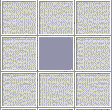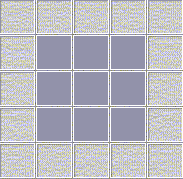Buildings and trees provide shade. You may calculate the number of hours of shade provided by these items to various squares in your layout. To keep things simple, we will assume that the sun travels directly overhead from east to west. The amount of shade that a tree provides depends upon the height of the tree and whether or not the tree is deciduous or coniferous. The amount of shade a building provides depends upon the side of the building to which you are adjacent.

Each square will have a shade rating. The rating depends on the amount of shade per day that a square gets. We will assume that there are 14 hours of sun per day during the growing season. Here are the three shade ratings:

 Shade Rating Hours of Shade per Day Full Sun < 2 Partial Sun 2 to 6 Shade > 6

If there are no buildings or trees providing shade to a square, that square will have 0 hours of shade and therefore, a rating of Full Sun.

If the shade rating for a square is Shade, the water requirements for that square will go down one level (e.g. Medium to Low)

## Buildings

The edge shadow cast by a building starts 3 squares west of the building and moves one square east every two hours. There is no shadow cast by the building during the middle two hours of the day.

## Trees

The canopy of a tree depends on what type of tree it is (coniferous or deciduous) and the height of the tree. The canopy is how far out the branches reach from the trunk.

 Type & Height of Tree Canopy Coniferous, < 7 m 1 square Coniferous, > 7 m 9 squares Deciduous, < 3 m 1 square Deciduous, 3 to 8 m 9 squares Deciduous, > 8 m 25 squares

If the canopy is only 1 square (see Figure 1 below), then that means the tree offers no shade to surrounding squares in the middle of the day.
Figure 1If the canopy is 9 squares (see Figure 2 below), then that means the tree offers shade to the surrounding 8 squares in a block as well as to its own square, in the middle of the day.
Figure 2Throughout the day, the centre of the shadow cast by a tree canopy for a tree that is less than 5 meters high starts 3 squares west of the tree and moves one square east every two hours. All the squares directly below the canopy are shaded for the middle two hours of the day.

Throughout the day, the centre of the shadow cast by a tree canopy for a tree that is 5 meters high or taller starts 6 squares west of the tree and moves one square east every hour. All the squares directly below the canopy are shaded for the middle two hours of the day.